# fcontour

•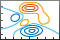## Syntax

fcontour(f)
fcontour(f,xyinterval)
fcontour(___,LineSpec)
fcontour(___,Name,Value)
fcontour(ax,___)
fc = fcontour(___)

## Description

example

fcontour(f) plots the contour lines of the function z = f(x,y) for constant levels of z over the default interval [-5 5] for x and y.

example

fcontour(f,xyinterval) plots over the specified interval. To use the same interval for both x and y, specify xyinterval as a two-element vector of the form [min max]. To use different intervals, specify a four-element vector of the form [xmin xmax ymin ymax].
fcontour(___,LineSpec) sets the line style and color for the contour lines. For example, '-r' specifies red lines. Use this option after any of the previous input argument combinations.

example

fcontour(___,Name,Value) specifies line properties using one or more name-value pair arguments.
fcontour(ax,___) plots into the axes specified by ax instead of the current axes.

example

fc = fcontour(___) returns a FunctionContour object. Use fc to query and modify properties of a specific FunctionContour object. For a list of properties, see FunctionContour Properties.

## Examples

collapse all

Plot the contours of $f\left(x,y\right)=\mathrm{sin}\left(x\right)+\mathrm{cos}\left(y\right)$ over the default interval of $-5 and $-5.

f = @(x,y) sin(x) + cos(y); fcontour(f)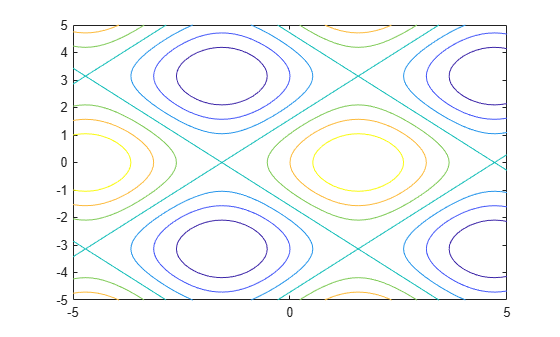Specify the plotting interval as the second argument of fcontour. When you plot multiple inputs over different intervals in the same axes, the axis limits adjust to display all the data. This behavior lets you plot piecewise inputs.

Plot the piecewise input

$\begin{array}{cc}erf\left(x\right)+\mathrm{cos}\left(y\right)& -5

over $-5.

fcontour(@(x,y) erf(x) + cos(y),[-5 0 -5 5]) hold on fcontour(@(x,y) sin(x) + cos(y),[0 5 -5 5]) hold off grid on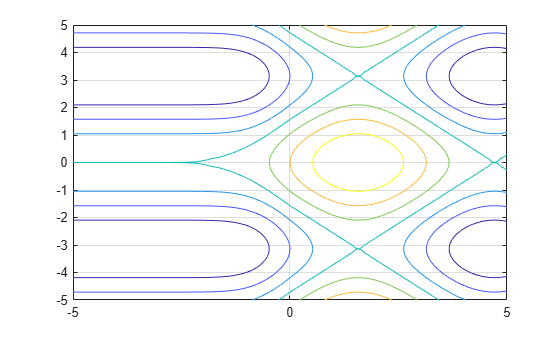Plot the contours of ${x}^{2}-{y}^{2}$ as dashed lines with a line width of 2.

f = @(x,y) x.^2 - y.^2; fcontour(f,'--','LineWidth',2)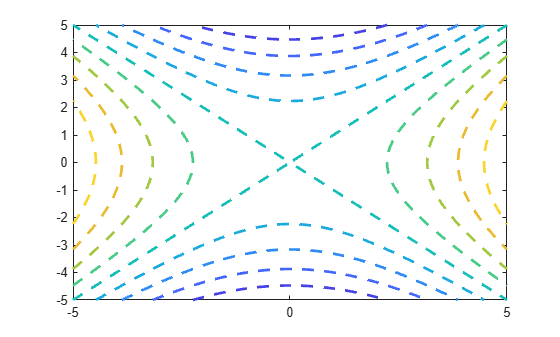Plot $\mathrm{sin}\left(x\right)+\mathrm{cos}\left(y\right)$ and $x-y$ on the same axes by using hold on.

fcontour(@(x,y) sin(x)+cos(y)) hold on fcontour(@(x,y) x-y) hold off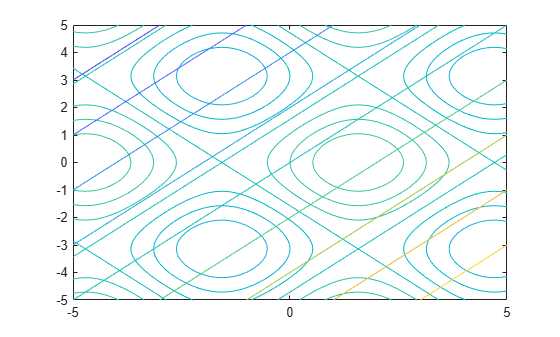Plot the contours of ${e}^{-\left(x/3{\right)}^{2}-\left(y/3{\right)}^{2}}+{e}^{-\left(x+2{\right)}^{2}-\left(y+2{\right)}^{2}}$. Assign the function contour object to a variable.

f = @(x,y) exp(-(x/3).^2-(y/3).^2) + exp(-(x+2).^2-(y+2).^2); fc = fcontour(f)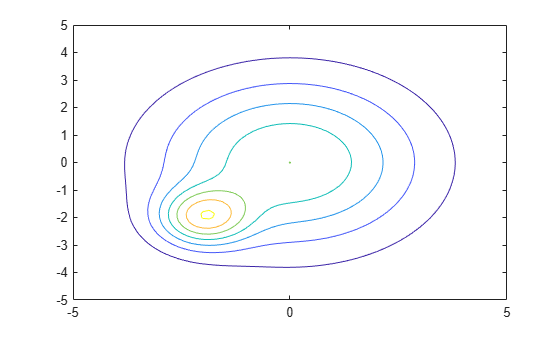fc = FunctionContour with properties: Function: @(x,y)exp(-(x/3).^2-(y/3).^2)+exp(-(x+2).^2-(y+2).^2) LineColor: 'flat' LineStyle: '-' LineWidth: 0.5000 Fill: off LevelList: [0.2000 0.4000 0.6000 0.8000 1 1.2000 1.4000] Show all properties 

Change the line width to 1 and the line style to a dashed line by using dot notation to set properties of the function contour object. Show contours close to 0 and 1 by setting the LevelList property. Add a colorbar.

fc.LineWidth = 1; fc.LineStyle = '--'; fc.LevelList = [1 0.9 0.8 0.2 0.1]; colorbar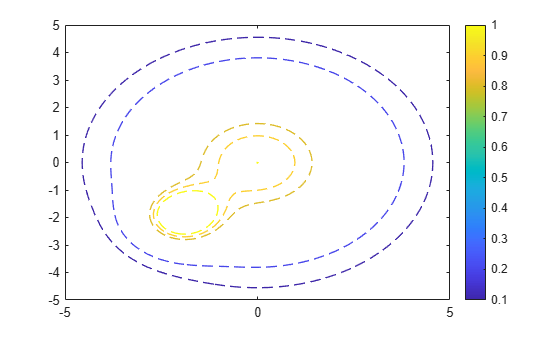Create a plot that looks like a sunset by filling the area between the contours of

$erf\left(\left(y+2{\right)}^{3}\right)-{e}^{\left(-0.65\left(\left(x-2{\right)}^{2}+\left(y-2{\right)}^{2}\right)\right)}.$

f = @(x,y) erf((y+2).^3) - exp(-0.65*((x-2).^2+(y-2).^2)); fcontour(f,'Fill','on');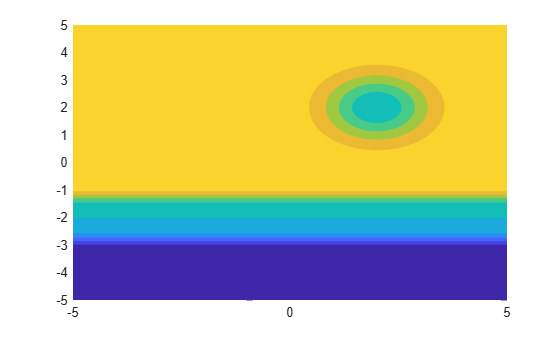If you want interpolated shading instead, use the fsurf function and set its 'EdgeColor' option to 'none' followed by the command view(0,90).

Set the values at which fcontour draws contours by using the 'LevelList' option.

f = @(x,y) sin(x) + cos(y); fcontour(f,'LevelList',[-1 0 1])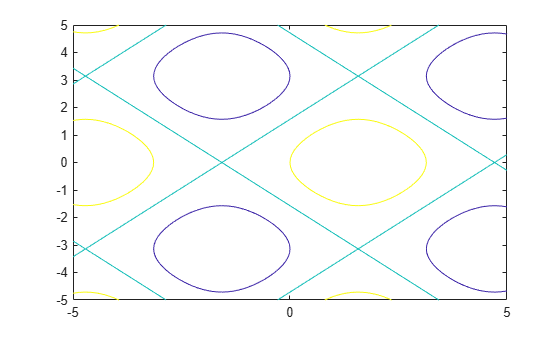Control the resolution of contour lines by using the 'MeshDensity' option. Increasing 'MeshDensity' can make smoother, more accurate plots, while decreasing it can increase plotting speed.

Create two plots in a 2-by-1 tiled chart layout. In the first plot, display the contours of $\mathrm{sin}\left(x\right)\mathrm{sin}\left(y\right)$. The corners of the squares do not meet. To fix this issue, increase 'MeshDensity' to 200 in the second plot. The corners now meet, showing that by increasing 'MeshDensity' you increase the resolution.

f = @(x,y) sin(x).*sin(y); tiledlayout(2,1) nexttile fcontour(f) title('Default Mesh Density (71)') nexttile fcontour(f,'MeshDensity',200) title('Custom Mesh Density (200)')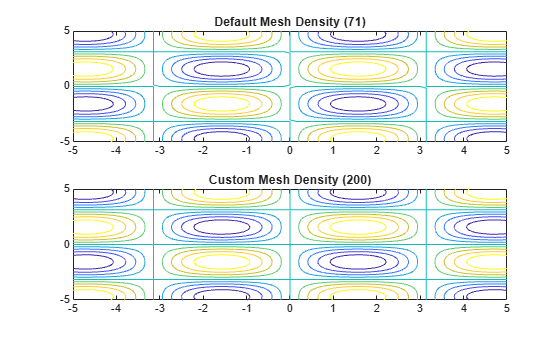Plot $x\mathrm{sin}\left(y\right)-y\mathrm{cos}\left(x\right)$. Display the grid lines, add a title, and add axis labels.

fcontour(@(x,y) x.*sin(y) - y.*cos(x), [-2*pi 2*pi], 'LineWidth', 2); grid on title({'xsin(y) - ycos(x)','-2\pi < x < 2\pi and -2\pi < y < 2\pi'}) xlabel('x') ylabel('y')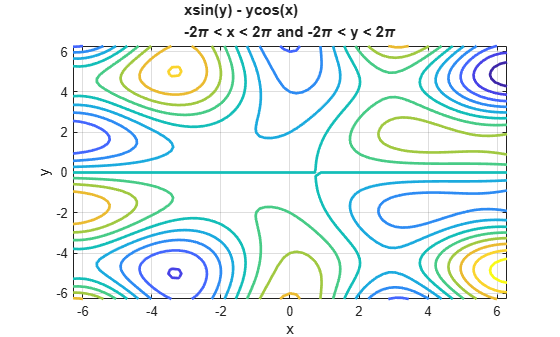Set the x-axis tick values and associated labels by setting the XTickLabel and XTick properties of the axes object. Access the axes object using gca. Similarly, set the y-axis tick values and associated labels.

ax = gca; ax.XTick = ax.XLim(1):pi/2:ax.XLim(2); ax.XTickLabel = {'-2\pi','-3\pi/2','-\pi','-\pi/2','0',... '\pi/2','\pi','3\pi/2','2\pi'}; ax.YTick = ax.YLim(1):pi/2:ax.YLim(2); ax.YTickLabel = {'-2\pi','-3\pi/2','-\pi','-\pi/2','0',... '\pi/2','\pi','3\pi/2','2\pi'};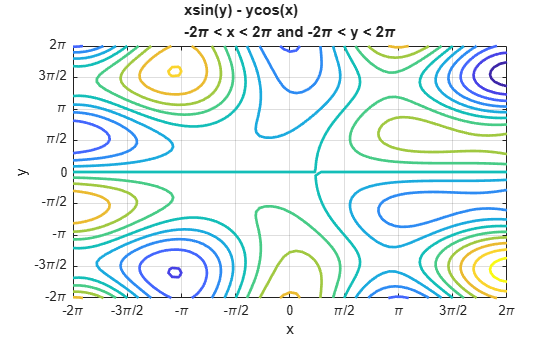## Input Arguments

collapse all

Function to plot, specified as a function handle to a named or anonymous function.

Specify a function of the form z = f(x,y). The function must accept two matrix input arguments and return a matrix output argument of the same size. Use array operators instead of matrix operators for the best performance. For example, use .* (times) instead of * (mtimes).

Example: f = @(x,y) sin(x) + cos(y);

Plotting interval for x and y, specified in one of these forms:

• Vector of form [min max] — Use the interval [min max] for both x and y.

• Vector of form [xmin xmax ymin ymax] — Use the interval [xmin xmax] for x and [ymin ymax] for y.

Axes object. If you do not specify an axes object, then the fcontour uses the current axes.

Line style and color, specified as a character vector or string containing a line style specifier, a color specifier, or both.

Example: '--r' specifies red dashed lines

These two tables list the line style and color options.

Line Style SpecifierDescription
-Solid line (default)
--Dashed line
:Dotted line
-.Dash-dot line
Color SpecifierDescription

y

yellow

m

magenta

c

cyan

r

red

g

green

b

blue

w

white

k

black

### Name-Value Arguments

Specify optional pairs of arguments as Name1=Value1,...,NameN=ValueN, where Name is the argument name and Value is the corresponding value. Name-value arguments must appear after other arguments, but the order of the pairs does not matter.

Before R2021a, use commas to separate each name and value, and enclose Name in quotes.

Example: 'MeshDensity',30

The properties listed here are only a subset. For a full list, see FunctionContour Properties.

Number of evaluation points per direction, specified as a number. The default is 71. Because fcontour uses adaptive evaluation, the actual number of evaluation points is greater.

Example: 30

Fill between contour lines, specified as 'on' or 'off', or as numeric or logical 1 (true) or 0 (false). A value of 'on' is equivalent to true, and 'off' is equivalent to false. Thus, you can use the value of this property as a logical value. The value is stored as an on/off logical value of type matlab.lang.OnOffSwitchState.

• A value of 'on' fill the spaces between contour lines with color.

• A value of 'off' leaves the spaces between the contour lines unfilled.

Contour levels, specified as a vector of z values. By default, the fcontour function chooses values that span the range of values in the ZData property.

Setting this property sets the associated mode property to manual.

Data Types: single | double | int8 | int16 | int32 | int64 | uint8 | uint16 | uint32 | uint64

Spacing between contour lines, specified as a scalar numeric value. For example, specify a value of 2 to draw contour lines at increments of 2. By default, LevelStep is determined by using the ZData values.

Setting this property sets the associated mode property to 'manual'.

Example: 3.4

Data Types: single | double | int8 | int16 | int32 | int64 | uint8 | uint16 | uint32 | uint64

Color of contour lines, specified as 'flat', an RGB triplet, a hexadecimal color code, a color name, or a short name. To use a different color for each contour line, specify 'flat'. The color is determined by the contour value of the line, the colormap, and the scaling of data values into the colormap. For more information on color scaling, see Control Colormap Limits.

To use the same color for all the contour lines, specify an RGB triplet, a hexadecimal color code, a color name, or a short name.

For a custom color, specify an RGB triplet or a hexadecimal color code.

• An RGB triplet is a three-element row vector whose elements specify the intensities of the red, green, and blue components of the color. The intensities must be in the range [0,1], for example, [0.4 0.6 0.7].

• A hexadecimal color code is a character vector or a string scalar that starts with a hash symbol (#) followed by three or six hexadecimal digits, which can range from 0 to F. The values are not case sensitive. Therefore, the color codes "#FF8800", "#ff8800", "#F80", and "#f80" are equivalent.

Alternatively, you can specify some common colors by name. This table lists the named color options, the equivalent RGB triplets, and hexadecimal color codes.

Color NameShort NameRGB TripletHexadecimal Color CodeAppearance
"red""r"[1 0 0]"#FF0000""green""g"[0 1 0]"#00FF00""blue""b"[0 0 1]"#0000FF""cyan" "c"[0 1 1]"#00FFFF""magenta""m"[1 0 1]"#FF00FF""yellow""y"[1 1 0]"#FFFF00""black""k"[0 0 0]"#000000""white""w"[1 1 1]"#FFFFFF""none"Not applicableNot applicableNot applicableNo color

Here are the RGB triplets and hexadecimal color codes for the default colors MATLAB® uses in many types of plots.

RGB TripletHexadecimal Color CodeAppearance
[0 0.4470 0.7410]"#0072BD"[0.8500 0.3250 0.0980]"#D95319"[0.9290 0.6940 0.1250]"#EDB120"[0.4940 0.1840 0.5560]"#7E2F8E"[0.4660 0.6740 0.1880]"#77AC30"[0.3010 0.7450 0.9330]"#4DBEEE"[0.6350 0.0780 0.1840]"#A2142F"Line width, specified as a positive value in points, where 1 point = 1/72 of an inch. If the line has markers, then the line width also affects the marker edges.

The line width cannot be thinner than the width of a pixel. If you set the line width to a value that is less than the width of a pixel on your system, the line displays as one pixel wide.

## Output Arguments

collapse all

One or more FunctionContour objects, returned as a scalar or a vector. You can use these objects to query and modify the properties of a specific contour plot. For a list of properties, see FunctionContour Properties.

## Version History

Introduced in R2016a